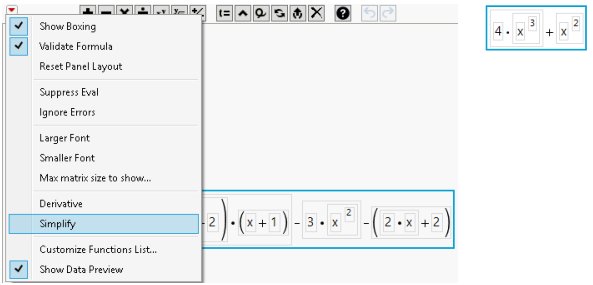Publication date: 04/12/2021

## Simplify Complex Formulas

When the Formula Editor contains a complex formula, JMP can simplify it using various algebraic rules. It can find constant expressions, distribute multiplication over addition, combine terms, and more.

To simplify complex formulas, follow these steps:

1. Select a portion or all of the formula.

2. Click the red triangle next to the keypad.

3. Select Simplify from the list as shown in Figure 7.16.

Figure 7.16 Selecting Simplify (Left) Produces a Simplified Formula (Right)Want more information? Have questions? Get answers in the JMP User Community (community.jmp.com).